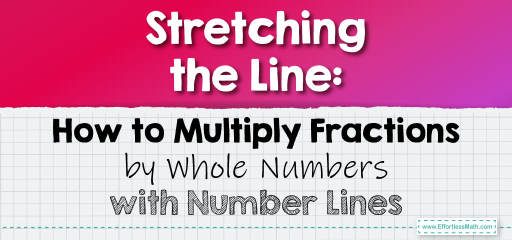# Stretching the Line: How to Multiply Fractions by Whole Numbers with Number Lines

Number lines offer a visual way to understand the multiplication of fractions by whole numbers.By representing fractions and whole numbers on a number line, we can visually see the product and gain a deeper understanding of the multiplication process. In this guide, we’ll explore how to use number lines to multiply fractions by whole numbers.

## Step-by-step Guide:

1. Setting Up the Number Line:

Draw a number line and mark it with appropriate intervals. If you’re multiplying a fraction by a whole number, the number line should extend at least up to that whole number.

2. Plotting the Fraction:

Mark the fraction you’re working with on the number line. For instance, if you’re multiplying $$\frac{1}{3}$$, mark a point one-third of the way between 0 and 1.

3. Multiplying Using Jumps:

To multiply the fraction by a whole number, make “jumps” on the number line equal to the size of the fraction. The number of jumps should be equal to the whole number you’re multiplying by.

4. Determining the Product:

The point where you land after making all the jumps represents the product of the fraction and the whole number.

5. Converting Improper Fractions:

If the result is an improper fraction (i.e., the numerator is greater than the denominator), convert it to a mixed number.

### Example 1:

Multiply $$\frac{1}{2}$$ by 3 using a number line.

Solution:

– Draw a number line from 0 to 3.

– Mark the point $$\frac{1}{2}$$ between 0 and 1.

– Make 3 jumps of size $$\frac{1}{2}$$.

After 3 jumps, you’ll land on the point 1.5 or $$1 \frac{1}{2}$$.

The Absolute Best Book for 5th Grade Students

### Example 2:

Multiply $$\frac{2}{3}$$ by 4 using a number line.

Solution:

– Draw a number line from 0 to 4.

– Mark the point $$\frac{2}{3}$$ between 0 and 1.

– Make 4 jumps of size $$\frac{2}{3}$$.

After 4 jumps, you’ll land on the point $$2 \frac{2}{3}$$.

### Practice Questions:

1. Multiply $$\frac{1}{4}$$ by 4 using a number line.

2. Multiply $$\frac{3}{5}$$ by 3 using a number line.

3. Multiply $$\frac{2}{6}$$ by 5 using a number line.

A Perfect Book for Grade 5 Math Word Problems!

1. 1

2. $$1 \frac{4}{5}$$ or 1.8

3. $$1 \frac{2}{3}$$ or 1.67

The Best Math Books for Elementary Students

### What people say about "Stretching the Line: How to Multiply Fractions by Whole Numbers with Number Lines - Effortless Math: We Help Students Learn to LOVE Mathematics"?

No one replied yet.

X
51% OFF

Limited time only!

Save Over 51%

SAVE $15 It was$29.99 now it is \$14.99